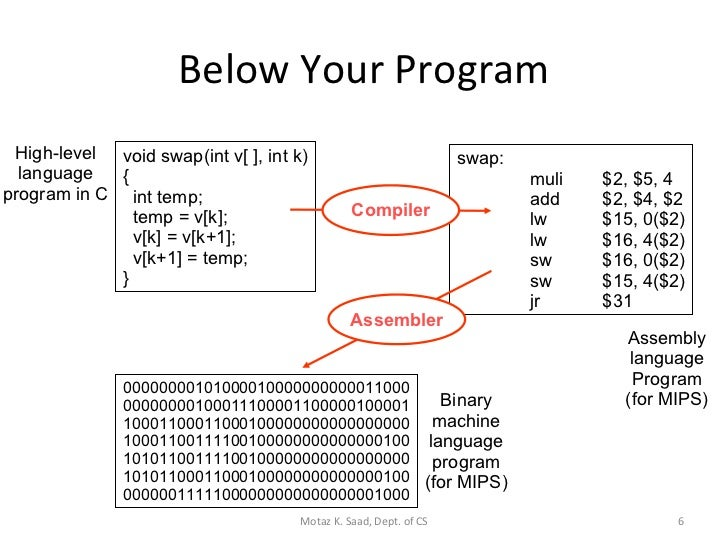Categories

# Assembler, Compiler, and Interpreter

How to convert C into binary codes.

This blog introduces the difference between these three concepts. Normally, we only consider compiler and assembler, but the idea of interpreter is often used as well.

Compiler takes higher-level languages such as C++ and Java, and turns them into assembly codes. Assembler turns the assembly codes into binary machine codes based on certain type of instruction set.

An interpreter looks like compiler, but it executes the codes right after compilation. For instance, the JVM Just-in-time interpreter can run some codes while compiling other parts. Interpreter is also more portable than compiler and used in Python, PHP, Perl, etc.

The code has to go through a preprocessor first in order to optimize the code structure, such as elimination of unused variables and empty function/blocks. The processed code then goes into compiler where the codes are turned into assembly codes (e.g. x86-64 or amdv8), then the assembly codes are turned into binary codes by the assembler, which is then executed by the central processor. The linker is used to link any external files/libraries that is imported in the original main.c file.An example of how the compiler and assembler turns codes into binaries

There are several aspects into the process of building a compiler or assembler. Here, we will talk about two of them: code parsing and optimization techniques.

In discrete math, the definition of a formal language is recursive. Any higher-level language is presumably a set of formal language, and can be parsed using their rules. Here, we use Haskell as an example to parse C into intermediate representations:

``````--C representation
1: fact(n) {
2: if (n == 0) {
3: \$return = 1
4: } else {
5: prod = 1
6: i=n
7: do {
8:        prod = prod * i
9: i = i + -1
10:     } while (i > 0)
11:     \$return = prod
12: }
13: }

type Function = (Id, [Id], Block)
type Block = [Statement]
data Statement = Assign Id Exp |
If Exp Block Block |
DoWhile Block Exp
deriving (Eq, Show)
data Exp = Const Int | Var Id | Apply Op Exp Exp | Phi Exp Exp
deriving (Eq, Show)
data Op = Add | Mul | Eq | Gtr
deriving (Eq, Show)

fact = ("fact",
["n"],
[If (Apply Eq (Var "n") (Const 0))
[Assign "\$return" (Const 1)]
[Assign "prod" (Const 1),
Assign "i" (Var "n"),
DoWhile
[Assign "prod" (Apply Mul (Var "prod") (Var "i")), Assign "i" (Apply Add (Var "i") (Const (-1)))
]
(Apply Gtr (Var "i") (Const 0)),
Assign "\$return" (Var "prod")
] ]
)``````

After parsing, we can then optimizing the code using several techniques, one of which is constant propagation in Static Single Assignment form. The idea is that we first translates codes in to Static Single Assignment form, which assign each variable only once. Thus, we can replace any occurrence of that variable directly with its value:

``````//Before optimization
1: basicBlock() {
2:    x0=1
3:    y0=2
4:    x1=x0+y0
5:    y1=x1*3
6:    \$return = y1
7: }

//After optimization
1: basicBlock() {
2:    x1 = 1 + 2
3:    y1 = x1 * 3
4:    \$return = y1
5: }``````

Here, we replaced x0 and y0 with its own value, thus saves time during execution.

There are many other optimization techniques, such as changing while loops to do-while loops(easier to execute in terms of instruction set), data-flow optimizations, etc. For more, visit specific techniques of compiler optimization.